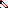# MINLPLib

### A Library of Mixed-Integer and Continuous Nonlinear Programming Instances

#### Instance: ex9_2_5

 Formatsⓘ ams gms lp mod nl osil pip Primal Boundsⓘ 9.00000000 p1 ( gdx sol ) (infeas: 2e-15)5.00000015 p2 ( gdx sol ) (infeas: 9e-16) Dual Boundsⓘ -0.00000048 (ANTIGONE)4.99999999 (BARON)5.00000000 (COUENNE)5.00000000 (LINDO)4.99999978 (SCIP) Referencesⓘ Floudas, C A, Pardalos, Panos M, Adjiman, C S, Esposito, W R, Gumus, Zeynep H, Harding, S T, Klepeis, John L, Meyer, Clifford A, and Schweiger, C A, Handbook of Test Problems in Local and Global Optimization, Kluwer Academic Publishers, 1999.Clark, P A and Westerberg, A W, Bilevel Programming for Steady-State Chemical Process Design - I. Fundamentals and Algorithms, Computers and Chemical Engineering, 14:1, 1990, 87-97. Sourceⓘ Test Problem ex9.2.5 of Chapter 9 of Floudas e.a. handbook Added to libraryⓘ 31 Jul 2001 Problem typeⓘ QCQP #Variablesⓘ 8 #Binary Variablesⓘ 0 #Integer Variablesⓘ 0 #Nonlinear Variablesⓘ 8 #Nonlinear Binary Variablesⓘ 0 #Nonlinear Integer Variablesⓘ 0 Objective Senseⓘ min Objective typeⓘ quadratic Objective curvatureⓘ convex #Nonzeros in Objectiveⓘ 2 #Nonlinear Nonzeros in Objectiveⓘ 2 #Constraintsⓘ 7 #Linear Constraintsⓘ 4 #Quadratic Constraintsⓘ 3 #Polynomial Constraintsⓘ 0 #Signomial Constraintsⓘ 0 #General Nonlinear Constraintsⓘ 0 Operands in Gen. Nonlin. Functionsⓘ Constraints curvatureⓘ indefinite #Nonzeros in Jacobianⓘ 19 #Nonlinear Nonzeros in Jacobianⓘ 6 #Nonzeros in (Upper-Left) Hessian of Lagrangianⓘ 8 #Nonzeros in Diagonal of Hessian of Lagrangianⓘ 2 #Blocks in Hessian of Lagrangianⓘ 5 Minimal blocksize in Hessian of Lagrangianⓘ 1 Maximal blocksize in Hessian of Lagrangianⓘ 2 Average blocksize in Hessian of Lagrangianⓘ 1.6 #Semicontinuitiesⓘ 0 #Nonlinear Semicontinuitiesⓘ 0 #SOS type 1ⓘ 0 #SOS type 2ⓘ 0 Infeasibility of initial pointⓘ 14 Sparsity JacobianⓘSparsity Hessian of Lagrangianⓘ```\$offlisting
*
*  Equation counts
*      Total        E        G        L        N        X        C        B
*          8        8        0        0        0        0        0        0
*
*  Variable counts
*                   x        b        i      s1s      s2s       sc       si
*      Total     cont   binary  integer     sos1     sos2    scont     sint
*          9        9        0        0        0        0        0        0
*  FX      0
*
*  Nonzero counts
*      Total    const       NL      DLL
*         22       14        8        0
*
*  Solve m using NLP minimizing objvar;

Variables  x1,objvar,x3,x4,x5,x6,x7,x8,x9;

Positive Variables  x3,x4,x5,x6,x7,x8,x9;

Equations  e1,e2,e3,e4,e5,e6,e7,e8;

e1.. (-3 + x3)*(-3 + x3) + (-2 + x1)*(-2 + x1) - objvar =E= 0;

e2..    x1 - 2*x3 + x4 =E= 1;

e3..  - 2*x1 + x3 + x5 =E= 2;

e4..    2*x1 + x3 + x6 =E= 14;

e5.. x4*x7 =E= 0;

e6.. x5*x8 =E= 0;

e7.. x6*x9 =E= 0;

e8..    2*x1 + x7 - 2*x8 + 2*x9 =E= 10;

* set non-default bounds
x3.up = 8;

Model m / all /;

m.limrow=0; m.limcol=0;
m.tolproj=0.0;

\$if NOT '%gams.u1%' == '' \$include '%gams.u1%'

\$if not set NLP \$set NLP NLP
Solve m using %NLP% minimizing objvar;

```

Last updated: 2019-02-14 Git hash: a71254dc